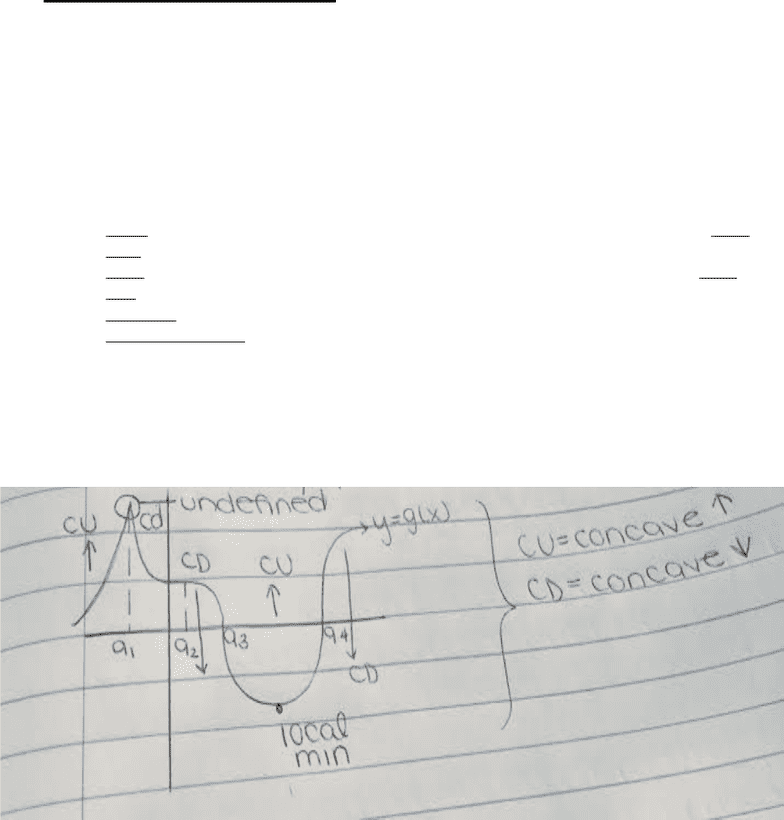# MATA32H3 Final: Final Exam - Fall 2017

155 views31 pages
University of Toronto
Scarborough
MATA32H3
Calculus for Management I
Fall 2017
Final Exam
Exam Guide
Unlock document

This preview shows pages 1-3 of the document.
Unlock all 31 pages and 3 million more documents.

CONTENTS
Exam Guide Covers the Following Topics:
Derivatives and Concavity
Absolute Extrema on a Closed and Bounded Interval
Horizontal &Vertical Asymptotes
Applied Optimization Problems
Integration
Integration by Parts
The Definite Integral
The Fundamental Theorem of Calculus
Properties of the Definite Integral
Applications of Integral to Area
Areas Bounded Between Curves
Unlock document

This preview shows pages 1-3 of the document.
Unlock all 31 pages and 3 million more documents.1st Derivative Test
Idea: given y=f(x), the 1st derivative is used to determine whether a critical number of a
function is a local max, min, or neither
*** Critical number is 0 or undefined
Let y=f(x) be a function and assume ‘c’ is a critical number of ‘y’. Assume (I) is an open
interval
1. Max: if f’(x) changes signs from + to -, as ‘x’ moves through I, the ‘f’ has a local
max at ‘c’
2. Min: If f’(x) changes sign from + to -, as ‘x’ moves through I, the ‘f’ has a local
min at ‘c’
3. Neither: if f’(x) does not change sign for ‘x’ to the left side of ‘c’ in I, then ‘f’ has
no local max/min at ‘c’
2nd Derivative
Gives information about the bending upwards/downwards of a function
(i.e. concavity (point of inflection)
Concavity Test
Suppose we have a function y=f(x) so the f’’(x) is defined on a given open interval (a,b)
!I
1. if f’’(x) > 0 for all x of I, the f(x) is concave up on I
2. If f’’(x) <0 for all x of I, then f(x) is concave down on I
We obtain intervals I, as in the concavity test
1. Find f’’(x)
2. Solve f’(x)=0
3. See if f’’(x) is ever undefined
Derivatives and Concavity
Unlock document

This preview shows pages 1-3 of the document.
Unlock all 31 pages and 3 million more documents.